# Programming Reference:TrialStatistics Class

## Location

BCI2000/src/shared//modules/application/utils

## Synopsis

The TrialStatistics class provides bookkeeping for trial outcomes in form of a frequency matrix recording target and result of each trial.

The TrialStatistics class is a descendant of the EnvironmentExtension class.

## Properties

### int Hits (r)

The number of trials where the result matched the target.

### int Total (r)

The total number of trials recorded.

### int Invalid (r)

The number of trials recorded as "invalid". Invalid trials do not enter into performance computation.

### float Bits (r)

The amount of information transferred since the last reset, given in bits. See the notes below for details.

## Events

### Initialize

During system initialization, TrialStatistics uses the NumberTargets parameter to determine the size of its internal frequency matrix.

## Methods

### Reset()

Resets the invalid trials counter, and all entries of the frequency matrix to 0.

### Update(int TargetCode, int ResultCode)

Adds the outcome of a trial to the frequency matrix.

### UpdateInvalid()

Increments the invalid trials counter.

## Remarks

The Bits() function computes the amount of information transferred through a noisy channel when for each combination of input and output symbols (target and result codes) the number of occurrences is given in form of the elements of a frequency matrix.

The computation uses the formula for information transfer through a noisy channel as given in Shannon, A Mathematical Theory of Communication, in: The Bell System Technical Journal, Vol. 27, 1948, section 16: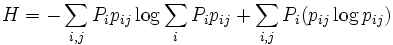$H = - \sum_{i,j}{P_i p_{ij}} \log\sum_i{P_i p_{ij}} + \sum_{i,j}{P_i (p_{ij} \log{p_{ij}})}$ where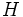$H$ is the information transferred per symbol,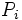$P_i$ is a probability distribution over input symbols, and noise is represented as a transition matrix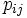$p_{ij}$ that contains the probability for output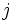$j$ if the input is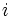$i$.

In the expression, the first term represents the entropy of the distribution of output symbols (result codes); the second term represents the reduction of this entropy introduced by noise, and is given as the negative of the entropy for each$p_{ij}$ row: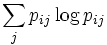$\sum_j p_{ij}\log p_{ij}$, weighted by the probability$P_i$ for the occurrence of its input symbol, i.e. for its actual occurrence during transmission.

We consider the elements of the frequency matrix as an estimate for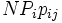$N P_i p_{ij}$ where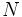$N$ is the number of symbols transferred, i.e. the number of trials, and the relative frequencies of target codes as an estimate for the$P_i$. From this, we compute the total number of bits transferred, i.e. Shannon's expression multiplied by$N$.

## Limitations

Shannon's expression is based on assumptions that are likely to be violated in practice, most notably

• the assumption that successive input symbols (target codes) are independent of each other -- this is clearly violated by obtaining target codes from a block randomization scheme, implying an over-estimate of the bit rate, but also
• the assumption that noise affects successive input independently is not necessarily fulfilled, especially in the presence of an adaptive algorithm.